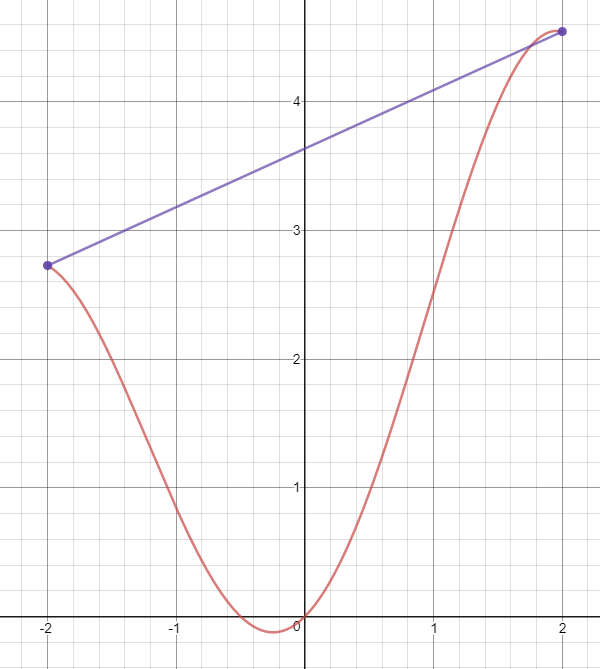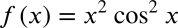Exit Ticket for Day 27 of Calculus
Please select your name from the following list: *
1. According to Rolle's Theorem, if f(x₁)=f(x₂), ______.
2. The Mean Value Theorem only applies to a function f(x) over some domain A if ______.
3. Look at the graph shown below. In red, I've drawn some function, and in purple I have connected the endpoints of this function on the domain [-2,2]. What does the Mean Value Theorem tell us about this situation?These are review questions:
4. What is the derivative of this function?5. Find the derivative of the following function:This form was created inside of Evansville Vanderburgh School Corporation. Report Abuse - Terms of Service